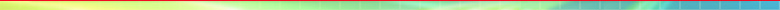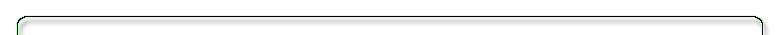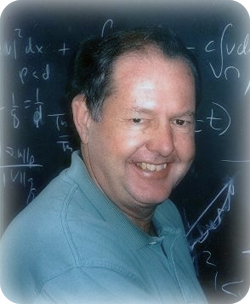Giles Auchmuty Professor of Mathematics - University of Houston Office Location: PGH 696 Telephone: (713) 743-3475 Fax: (713) 743-3505 E-mail: auchmuty@uh.edu Physical, and mail, address is University of Houston Department of Mathematics 4800 Calhoun Ave, Houston, TX 77204-3008

### Research Interests:

Currently my research centers on the properties of Steklov eigenproblems for linear elliptic operators and  their applications in a range of areas including the equations of fluid mechanics, approximation theory, numerical analysis and gravitational field theories.  A particular interest is how solutions of various problems depend on boundary data and their application to  solving inverse problems.

Steklov eigenproblems are eigenproblems for partial differential equations where the eigenvalue appears only in the boundary conditions.  They are important for studying boundary conditions and trace theorems for elliptic problems; the eigenvalues provide best constants in important inequalities. They provide analytic approximations to solutions of various boundary value problems. An important recent result is the construction of the singular value decomposition for the  Poisson kernel and of Reproducing Kernels for certain spaces of harmonic functions.

Another current interest is the question of what are well-posed boundary value problems for various equations in classical field theories.  These includes div-curl systems, Stokes equations and Maxwell's equations. The issues center on what boundary conditions are required for the equations to be well-posed and how can the solutions be represented? Motivations for much of this work comes from problems in classical fluid mechanics, electromagnetic field theories and geophysical problems where the solutions are determined as much by the boundary conditions as by the equations.

Most of this  is theoretical mathematics, involving convex and real  analysis, partial differential equations and variational principles. I have many interesting, open questions that would benefit from more computational study - including issues such as efficient ways to solve boundary value problems for the Laplacian on polygons. Students interested in working on these problems are welcome to come and discuss possible projects.  You should have a good grounding in real analysis or computational work.

For a full listing of research papers, arranged by topic, see Scientific Papers.
This research has been supported for over 35 years by NSF grants, for 9 years by Robert A Welch foundation awards,  and by a number  of other awards. See  also the Google Scholar  listing.
or Reviews from    MathSciNet.

### Research Students and Projects:

Here is a listing of  Ph.D. graduates whose theses I  have supervised. I have also supervised many M.S. theses and some undergraduate honors projects on a variety of mathematical  topics.

### Editorial:

Currently I am on the editorial board of  Numerical Functional Analysis and Optimization and of Nonlinear Analysis and Differential Equations, published by Hikari Press.

Current Address: Department of Mathematics, PGH Building, University of Houston, Houston, Texas 77204-3008
Phone(UH): (713) 743-3500 - Fax(UH): (713) 743-3505